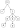# Trie Implementation in C#

## Basic#

Trie, sometimes also called `Prefix Tree`, is a data structure that stores every character of words in a Tree-style structure. The most popular application of Trie is Autocomplete system which is commonly found in search engine and IDE.

Trie consists of `node` that contains characters where if we traverse from the first character (root) to the leaf will form a word (string).

In below example, there are four words which are `A`,`tea`,`the` and `to`. A node (character) can have many children (characters). Each node also has `IsEndOfWord` property to mark whether the node is the end of a word (leaf) or not.``````public class TrieNode
{
public Dictionary<char, TrieNode> Children { get; set; }
public bool IsEndOfWord { get; set; }

public TrieNode()
{
Children = new Dictionary<char, TrieNode>();
IsEndOfWord = false;
}
}
``````

``````public class Trie
{
public TrieNode Root { get; }

public Trie(TrieNode trieNode)
{
Root = trieNode;
}

// Code to insert a word

// Code to search a word

// Code to delete a word

// Etc.
}
``````

## Implementation#

### Insertion#

The algorithm to insert a word in a Trie:

1. Set root as current node
2. Iterating each character of the word
3. If children (dictionary) doesn’t contain the character, then add the character to current children
4. Get the node of current character and assign it to current node so that current node will point to the next character
5. Repeat step number 3 until finish iterating the word
6. Mark current node as a leaf by setting `IsEndOfWord` to be true

The complexity of this operation is O(n) where n is the length of the word.

``````public void Insert(string word)
{
TrieNode current = Root;
foreach (char ch in word)
{
if (!current.Children.ContainsKey(ch))
{
}
current = current.Children[ch];
}
current.IsEndOfWord = true;
}
``````

### Deletion#

To delete a word, we need to follow these steps:

1. Recursively traversing the Trie down to the leaf
2. If in the middle, current character is not in children, it means the word does not exist, we can return immediately
3. If we reach the end of the word but `IsEndOfWord` is not marked as the end of the word, it means it’s also not a valid word, we can return immediately.
4. Otherwise, we are now at the leaf. We can return and delete the leaf.

The complexity of this operation is O(n) where n is the length of the word.

``````public void Delete(string word)
{
Delete(Root, word, 0);
}

private Boolean Delete(TrieNode current, string word, int index)
{
if (index == word.Length)
{
if (!current.IsEndOfWord)
{
return false;
}
current.IsEndOfWord = false;
return current.Children.Count == 0;
}
if (!current.Children.TryGetValue(word[index], out TrieNode node))
{
return false;
}
bool shouldDeleteCurrentNode = Delete(node, word, index + 1) && !node.IsEndOfWord;
if (shouldDeleteCurrentNode)
{
current.Children.Remove(word[index]);
return current.Children.Count == 0;
}
return false;
}
``````

### Searching#

To search a word, we need to follow these steps:

1. Iterate each character of the word
2. If current node’s children do not contain the character, the word is not valid. We can return immediately. Otherwise, we should have reference to the next character’s node.
3. Set current node to refer to the next character’s node from previous step.
4. return `IsEndOfWord` to confirm whether we are on the leaf which means it is a word. Thus, we have found the word.

The complexity of this operation is O(n) where n is the length of the word.

``````public bool Search(string word)
{
TrieNode current = Root;
foreach (char ch in word)
{
if (!current.Children.TryGetValue(ch, out TrieNode node))
{
return false;
}
current = node;
}

return current.IsEndOfWord;
}
``````

### StartsWith#

The implementation of `StartsWith` operation is the same with `Search` operation, except that in this operation we treat prefix as a word.

After we stop iteration, the last node will contain all nodes that will lead to all words that start with the prefix.

The complexity of `StartsWith` operation is O(n), but if we need to collect all the words, it will depend on the number of characters that we have after the prefix.

``````public List<string> StartsWith(string prefix)
{
List<string> result = new List<string>();

TrieNode current = Root;
foreach (char ch in prefix)
{
if (!current.Children.TryGetValue(ch, out TrieNode node))
{
return result;
}
current = node;
}

StringBuilder sbPrefix = new StringBuilder(prefix);
foreach (var pair in current.Children)
{
CreateStrings(sbPrefix.Append(pair.Key), pair, result);
sbPrefix.Remove(sbPrefix.Length - 1, 1);
}

return result;
}

private void CreateStrings(StringBuilder prefix, KeyValuePair<char, TrieNode> pair, List<string> result)
{
if (pair.Value.Children.Count == 0)
{